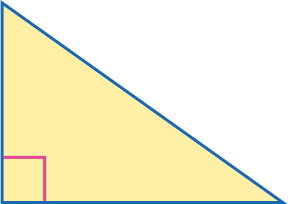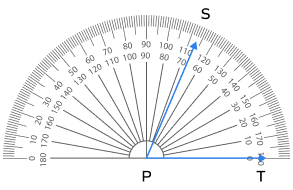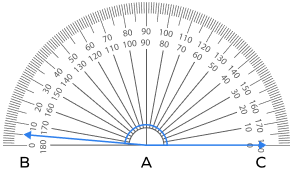# What is a Protractor – Definition with Examples

## What is a Protractor?

A protractor is a semicircular geometry tool divided into 180 equal parts, each measuring 1°. It is used for drawing angles of known measures and finding angles of unknown measures and is usually made of transparent plastic, glass, steel, or wood. The geometry protractor have two scales marked on it: outer and inner. The outer scale starts from 0° and goes up to 180° in a clockwise direction, and the inner scale has the same range in the counterclockwise direction.

## How To Measure an Angle Using a Protractor

Step 1: Place the center point of the protractor over the angle’s vertex.

Step 2: Adjust the protractor (without shifting the center from the vertex) so that one arm of the angle is along the baseline of the protractor.

Step 3: Look at the scale where the baseline arm points to 0 degrees.

Step 4: Read the measure of the angle where the other arm crosses the scale.

A protractor in math is a very useful tool for measuring angles of different shapes, such as triangles, squares, hexagons, and others.

## How Do You Draw an Angle Using a Protractor

You can follow the following steps for drawing an angle with a protractor.

Suppose you have to draw an angle of 50°.

1. Use a ruler to draw a horizontal line AB on the paper.
1. Take a protractor and place it such that its center point coincides with point A and its baseline coincides with AB.
1. Mark point C, where you find a 50° angle on the protractor.
1. Remove the protractor and join points C and A using a ruler.
1. You have drawn an angle of 50° using a protractor.

## Solved Examples On Protractor

Example 1: Find the measure of each angle of an equilateral triangle with the help of a protractor.

Solution: Align the protractor with the vertices of △ABC. Note the angle obtained in each case. You will find that all the angles of the equilateral triangle measure 60°.

Example 2: What will be the angle created at vertex B of the square?

Solution: Place the center of the protractor at vertex B with either AB or BC coinciding with the baseline and note the reading. You will get a 90° angle.

Example 3: What is the measure of the angle marked on the following protractor?

Solution: The side of the angle lies on the 100° marking of the protractor.

## Practice Problems On Protractor

1

### What is the measure of the angle shown in the following right triangle?45°
90°
60°
120°
CorrectIncorrect
A right angle always measures 90°. You can also use a protractor to confirm the measure.
2

### What is the measure of the angle shown by the protractor in the following figure?70°
65°
75°
67°
CorrectIncorrect
Observe the markings on the protractor closely. You will note that the side of the angle lies at 67°.
3

### What is the measure of the angle shown by the protractor in the following figure?180°
176°
170°
175°
CorrectIncorrect
Observe the reading on the protractor closely. You will note that the side of the angle aligns with the 175°.
4

### What is the measure of angle CAB given below?100°
105°
75°
120°
CorrectIncorrect